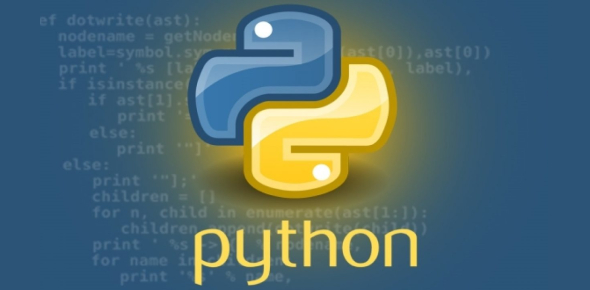# Python Programming Exam Quiz: Trivia

17 Questions | Total Attempts: 106SettingsPython programming is a general-purpose language that enables the programmer for web development, machine learning, operating systems, mobile application development and video games. In this quiz, you will get to test out how much you know about this language as well as how to write it. Do you think you know enough to answer all of them correctly? Do give it a try and get to find out!

• 1.
Python writes data on screen using which function?
• A.

Cout

• B.

(display )

• C.

Print()

• D.

Echo

• E.

Console.log()

• F.

System.out.println()

• 2.
Python can recognise this data: "hello"
• A.

Yes

• B.

No

• C.

Sometimes

• 3.
Python can recognise this data: 56.97
• A.

Yes

• B.

No

• C.

Sometimes

• 4.
Python can recognise this data:   computer
• A.

Yes

• B.

No

• C.

Sometimes

• 5.
Python can recognise this code: 3 + 6 * 8
• A.

Yes

• B.

No

• C.

Sometimes

• 6.
What does Python use to store values?
• 7.
Python will run this program without errors: print("hello")
• A.

True

• B.

False

• 8.
Python will run this program without errors: print(765.9908) print("that was a number")
• A.

True

• B.

False

• 9.
The output of this program will be? print("5+7+2")
• A.

14

• B.

12

• C.

5+7+2

• D.

Nothing. It will return an error.

• 10.
What will the output of this program be? print("Hello") text = "This is a number" print("Hi, " + text) number = 4 print(box + 2) print(box * 2) print(box * box - box)
• A.

Hello Hi, This is a number 6 8 12

• B.

HelloHi, This is a number6812

• C.

Nothing, it will return an error

• D.

Hello Hi, text box2 boxbox box

• E.

Hello This is a number Hi, 4 box2 boxbox boxbox-box

• 11.
This program will run without errors: print(x) x = 3 print(x+3)
• A.

True

• B.

False

• 12.
What structure do you use to let the computer make decisions in Python?
• A.

Because structure

• B.

If statement

• C.

Decision structure

• D.

Decide statement

• 13.
What optional part can you add to an if statement?
• A.

Who

• B.

Else

• C.

Then

• D.

Nothing

• 14.
Which of these could python run without errors?
• A.

X = 5 if x < 10 print("hi")

• B.

X = 5 if x < 10: print("hi")

• C.

Both

• D.

Neither

• 15.
This program is full of errors. What do you need to change to make it correct?cat_weight = 45 if cat_wieght = 50; print your cat is healthy else: print unhealthy
• A.

The variable name has been spelled wrong

• B.

• C.

If statements end in a colon, not semicolon

• D.

Instructions underneath an if and an else should be indented by 4 spaces

• E.

Every line ends in a semicolon

• F.

Words that python should understand should be "surrounded by quotes".

• G.

You have to say what type of data something is when you declare a variable.

• H.

Else statements end in a semicolon, not a colon

• I.

Print() should always be written with brackets

• J.

You should use ==, not = to check if two things are equal

• K.

You should always include units when working with numbers in Python

Related TopicsBack to top